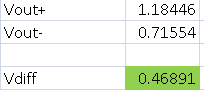This thread has been locked.

If you have a related question, please click the "Ask a related question" button in the top right corner. The newly created question will be automatically linked to this question.

# THS4503-EP: TINA TI DC analysis vs speadsheet using given equations

Part Number: THS4503-EP
Other Parts Discussed in Thread: THS4503, TINA-TI

Hi,
I am trying to analyze a simple circuit using TINA TI for THS4503. I find some discrepancy in verifying the DC analysis by TINA TI versus the calculations using the equations given in datasheet. I have attached a snapshot for the circuit under analysis for your reference.
Using equations for (1) for VOUT+ & (2) for VOUT- given in the datasheet, I get the following results. I need assistance to understand which one is correct and why.Thanks,

Pawan